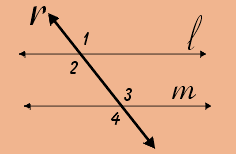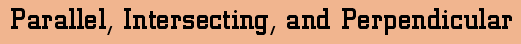For any two lines in the same plane, one of two relationships can occur: the lines will run parallel to each other, or they will intersect.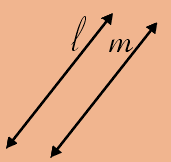Lines m and l are parallel lines. They will travel to infinity in either direction and never intersect.
 Lines x and y are intersecting lines. Lines can only intersect at one point and only one point.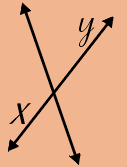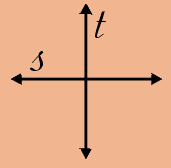When two lines intersect and form right angles, they are called perpendicular lines. Lines s and t are perpendicular lines. Vertical AnglesWhen two parallel lines are cut by another line (a transversal) angles of equal measure are created. The measures of L2 =L3, the measure of L1=L4. Angles 2 and 3 are called alternate interior angles. Angles 1 and 4 are called alternate exterior angles.